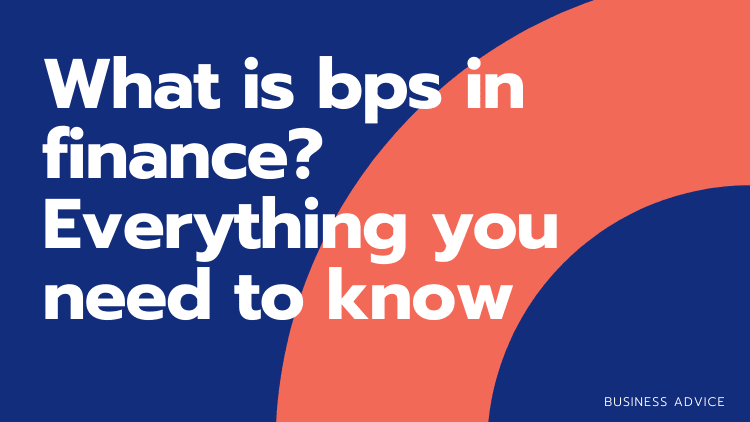# What is bps in finance? Everything you need to knowBps is a common abbreviation in finance for the term “basis points”, which are a smaller unit of measure for percentages. A basis point is equal to 1/100th of one percentage point, so 10 basis points are the same as 0.1%. This might seem like an insignificant amount, but even 1 bps can make a huge difference in large sums. It’s important to understand how this conversion works, because many investments are measured in terms of bps instead of percentage.

In this article, we will explain what basis points are, how they are used, and why they are so important in finance and investments.  We will also explain where the term basis point comes from, and why people use it instead of percentages.## What are basis points?

Basis points are the unit used to measure interest rates on loans, bonds and other financial products. They represent one-hundredth of a percentage point (.01%). The term is derived from the Latin word basis meaning “support” or “foundation.” A change in 100 basis points equals a change of 1% – which can also be written as +/-1%. For example, an increase in 200 bps would equal to +2%, whereas a decrease in 500 bps would equal -5%.

## What is calculated in bps?

The main use for basis point calculations is in finance and economics when people want to measure small changes over time.  For example, a change of one basis point in the cost-of-living index would be equivalent to an increase or decrease in inflation of 0.01%.

Another common use for bps is as part of the yield on a bond that pays periodic interest payments. For example, a £10,000 bond paying annual interest at 100 bps will pay £100 per year; while if it paid 200 bps, then it would pay £200 per year (a 50% higher rate).

Bps are also used to describe the changing interest rates of a home mortgage. This is because mortgages rates will often change less than 1%. You can fry your brain thinking about mortgage repayments increasing 0.35%, whereas using 35 bps is easier to compute.

## Why do people use bps instead of percentages?

As can be seen with the mortgage example, people often use bps because % calculations can quickly get confusing with smaller numbers. After all, what does 0.02% look like? Most of us think of percentages as being 0 to 100, so when we start getting into decimal points, it seems to defy the whole purpose of using percentages. Bps is more mentally digestible, and can even enable us to do seemingly difficult calculations in our heads.

## When are percentages and bps used at the same time?

When percentages and bps are used together, it is often to clarify an ambiguous change in circumstances.

For instance, if you had a savings account that paid 5% interest per year, and it then underwent a 5% boost, what does this mean?

Does it mean that you now receive 10% interest? (your original 5% + 5% boost)

Or does it mean that you now receive 5.25%? (your original 5% + 5% of 5)

In order to clear up the confusion, if we say that the account pays 5% interest and has received a 500bps increase, you know that you will now receive 10%.

What is the equation for converting bps into % and vice versa?

This equation is actually very simple once you are comfortable with the relationship between bps and %.

To convert % to bps, you just multiply by 100.

For example:

1% = 100bps

1.5% = 150bps

 SHARE: TAGS: